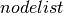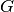Warning

This documents an unmaintained version of NetworkX. Please upgrade to a maintained version and see the current NetworkX documentation.

# to_numpy_recarray¶

to_numpy_recarray(G, nodelist=None, dtype=[('weight', <type 'float'>)], order=None)[source]

Return the graph adjacency matrix as a NumPy recarray.

Parameters: G (graph) – The NetworkX graph used to construct the NumPy matrix. nodelist (list, optional) – The rows and columns are ordered according to the nodes in. Ifis None, then the ordering is produced by G.nodes(). dtype (NumPy data-type, optional) – A valid NumPy named dtype used to initialize the NumPy recarray. The data type names are assumed to be keys in the graph edge attribute dictionary. order ({‘C’, ‘F’}, optional) – Whether to store multidimensional data in C- or Fortran-contiguous (row- or column-wise) order in memory. If None, then the NumPy default is used. M – The graph with specified edge data as a Numpy recarray NumPy recarray

Notes

Whendoes not contain every node in, the matrix is built from the subgraph ofthat is induced by the nodes in.

Examples

>>> G = nx.Graph()